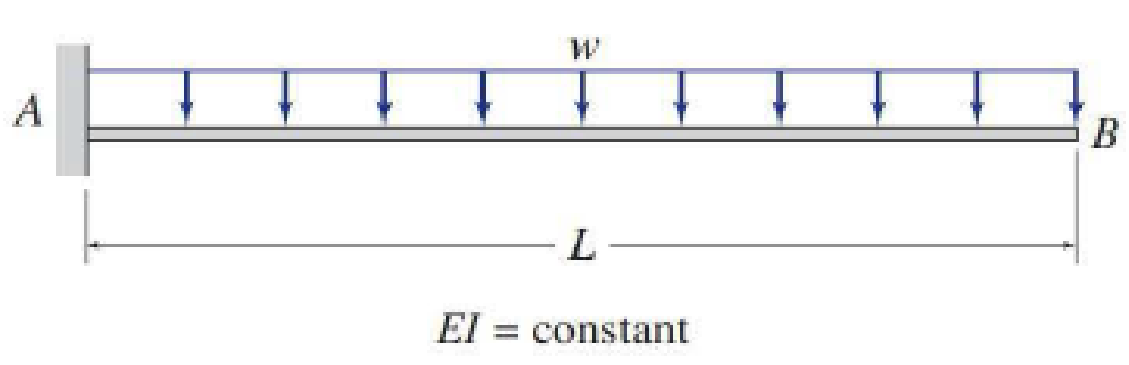# Use the conjugate-beam method to determine the slope and deflection at point B of the beams shown in Figs. P6.9 through P6.12. FIG. P6.10, P6.36

#### Solutions

Chapter
Section
Chapter 6, Problem 36P
Textbook Problem
189 views

## Use the conjugate-beam method to determine the slope and deflection at point B of the beams shown in Figs. P6.9 through P6.12.FIG. P6.10, P6.36

To determine

Find the slope θB and deflection ΔB at point B of the given beam using the conjugate-beam method.

### Explanation of Solution

Calculation:

Consider the flexural rigidity EI of the beam is constant.

Show the given beam as in Figure (1).

Consider upward is positive and downward is negative.

Consider clockwise is negative and counterclockwise is positive.

Determine the bending moment at point A using the relation;

MA(w×l×l2)=0

Here, w is the uniformly distributed load and l is the distance.

MA=wl22

Show the M/EI diagram for the given beam as in Figure (2).

Conjugate-beam method:

In the given beam system, point A is a fixed end and point B is free end. But in the conjugate-beam method the fixed end of a real beam becomes free and the free end of real beam changed into the fixed end.

Show the M/EI diagram for the conjugate-beam as in Figure (2).

Calculation of shear at B in the conjugate-beam:

The shear force at B of the conjugate beam is equal to the slope at B on the real beam.

Consider the external forces acting (left of B) upward on the free body diagram as positive.

Determine the shear force using the relation;

SB=(13×b×h)

Here, b is the width and h is the height of the triangle.

Substitute L for b and wL22EI for h

### Still sussing out bartleby?

Check out a sample textbook solution.

See a sample solution

#### The Solution to Your Study Problems

Bartleby provides explanations to thousands of textbook problems written by our experts, many with advanced degrees!

Get Started

Find more solutions based on key concepts
For Problems 16.24 through 16.28, using the rules discussed in this chapter, show the dimensions of the views s...

Engineering Fundamentals: An Introduction to Engineering (MindTap Course List)

Review the files and folders on your storage device. In your opinion, do you feel they are organized effectivel...

Enhanced Discovering Computers 2017 (Shelly Cashman Series) (MindTap Course List)

Describe two safety precautions you should observe when using layout dye.

Precision Machining Technology (MindTap Course List)

How is due diligence different from due care? Why are both important?

Principles of Information Security (MindTap Course List)

Write the SQL command to save the rows inserted in Problem 66.

Database Systems: Design, Implementation, & Management

What arc problems can occur in deep or narrow weld joints?

Welding: Principles and Applications (MindTap Course List)

If your motherboard supports ECC DDR3 memory, can you substitute non-ECC DDR3 memory?

A+ Guide to Hardware (Standalone Book) (MindTap Course List)